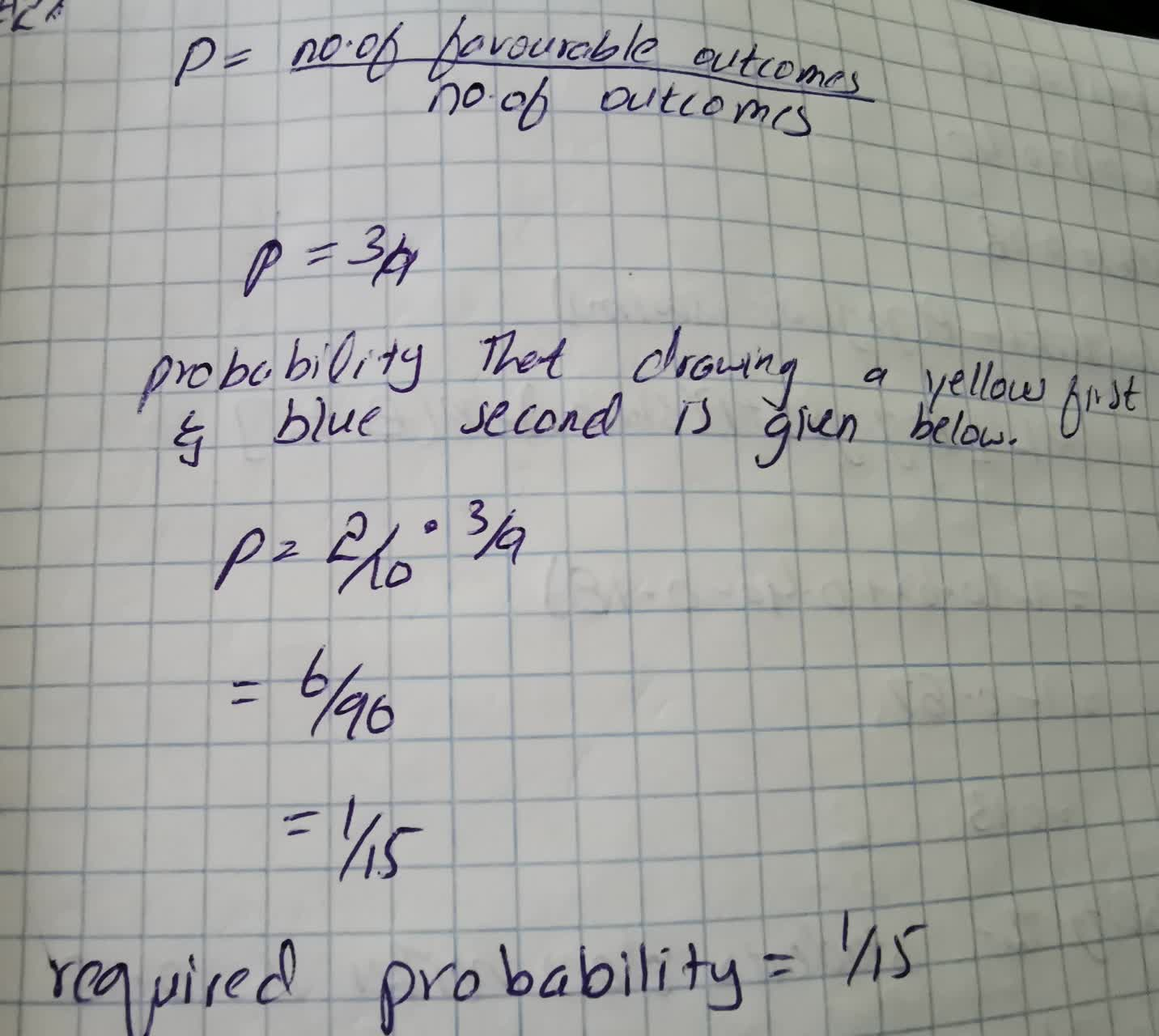# There are 10 balls in a bag: 4 red, 3 blue, 1 green, and 2 yellow. What is the probability that you will draw yellow first and blue second?cistG 2021-09-07 Answered
There are 10 balls in a bag: 4 red, 3 blue, 1 green, and 2 yellow. Answer each question by reporting probability as a simplified fraction, a decimal, or a percent. What is the probability that you will draw yellow first and blue second?
You can still ask an expert for help

## Want to know more about Probability?

• Questions are typically answered in as fast as 30 minutes

Solve your problem for the price of one coffee

• Math expert for every subject
• Pay only if we can solve itTalisha
The probability is